Mutual Information Joint Entropy Conditional Entropy Contents Entropy

• Slides: 13Mutual Information, Joint Entropy & Conditional Entropy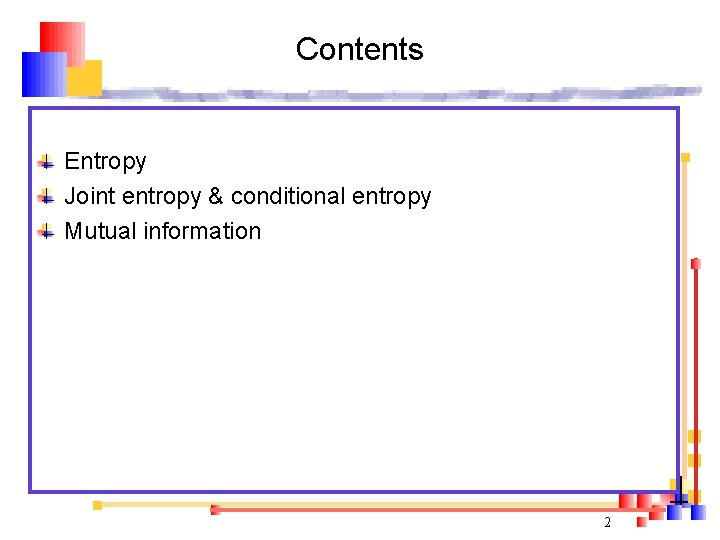Contents Entropy Joint entropy & conditional entropy Mutual information 2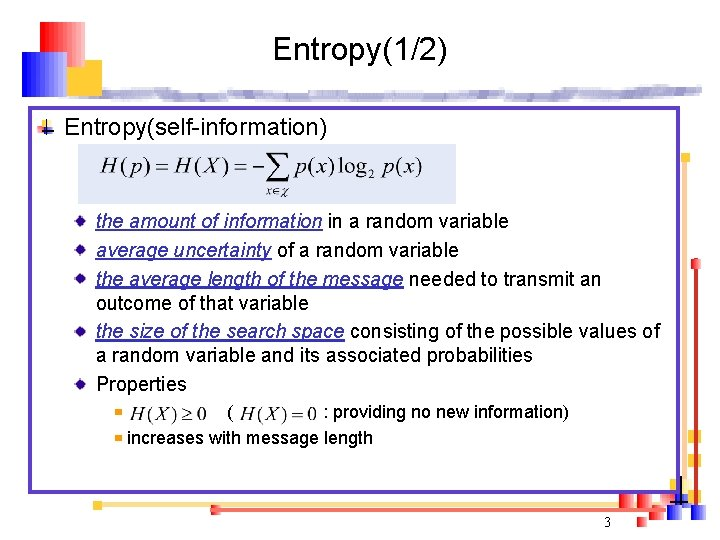Entropy(1/2) Entropy(self-information) the amount of information in a random variable average uncertainty of a random variable the average length of the message needed to transmit an outcome of that variable the size of the search space consisting of the possible values of a random variable and its associated probabilities Properties ( : providing no new information) increases with message length 3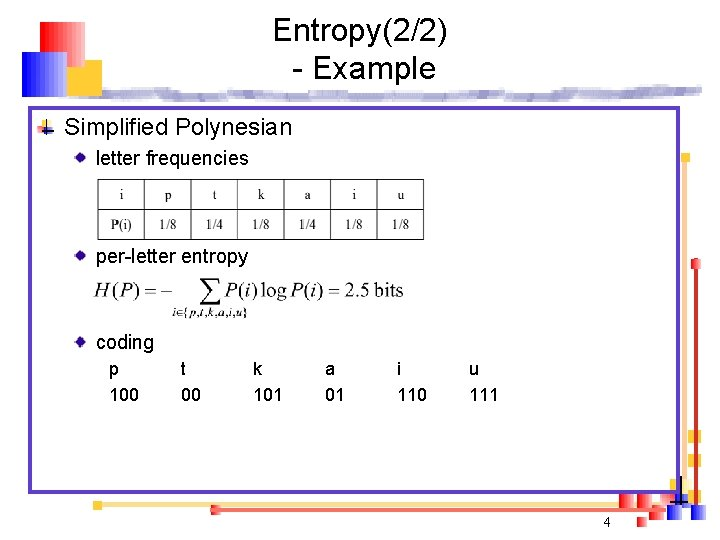Entropy(2/2) - Example Simplified Polynesian letter frequencies per-letter entropy coding p 100 t 00 k 101 a 01 i 110 u 111 4Joint Entropy & Conditional Entropy(1/4) Joint Entropy the amount of information needed on average to specify both their values Conditional Entropy how much extra information you still need to supply on average to communicate Y given that the other party knows X 5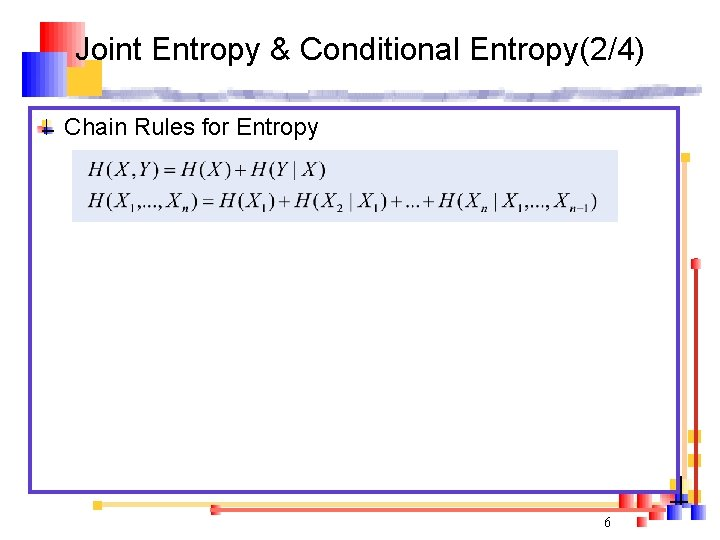Joint Entropy & Conditional Entropy(2/4) Chain Rules for Entropy 6Joint Entropy & Conditional Entropy(3/4) - Example Simplified Polynesian Revisited syllable structure all words consist of sequences of CV syllables. C: consonant, V: vowel 7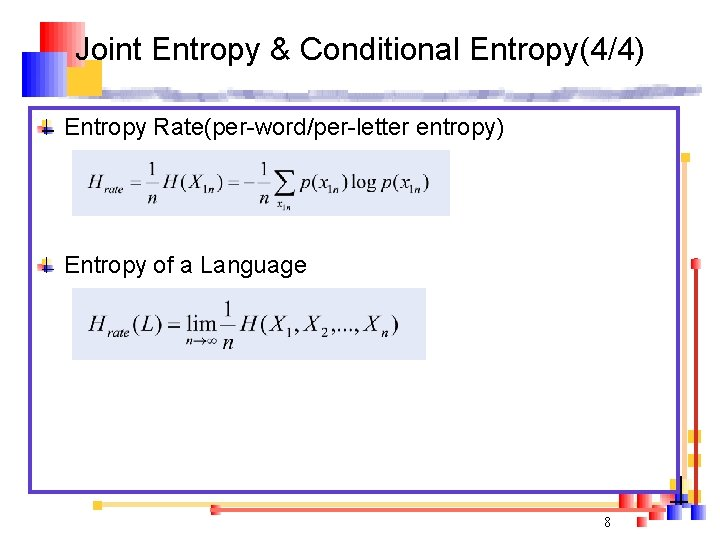Joint Entropy & Conditional Entropy(4/4) Entropy Rate(per-word/per-letter entropy) Entropy of a Language 8Mutual Information(1/2) Mutual Information the reduction in uncertainty of one random variable due to knowing about another the amount of information one random variable contains about another measure of independence : two variables are independent grows according to. . . the degree of dependence the entropy of the variables 9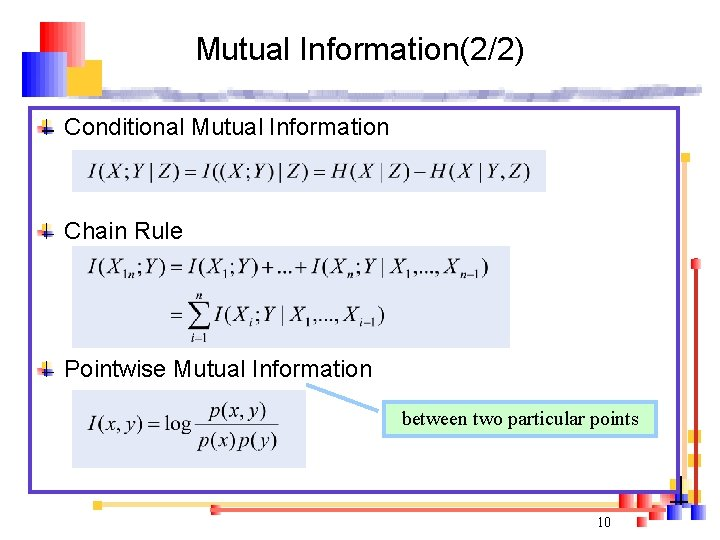Mutual Information(2/2) Conditional Mutual Information Chain Rule Pointwise Mutual Information between two particular points 10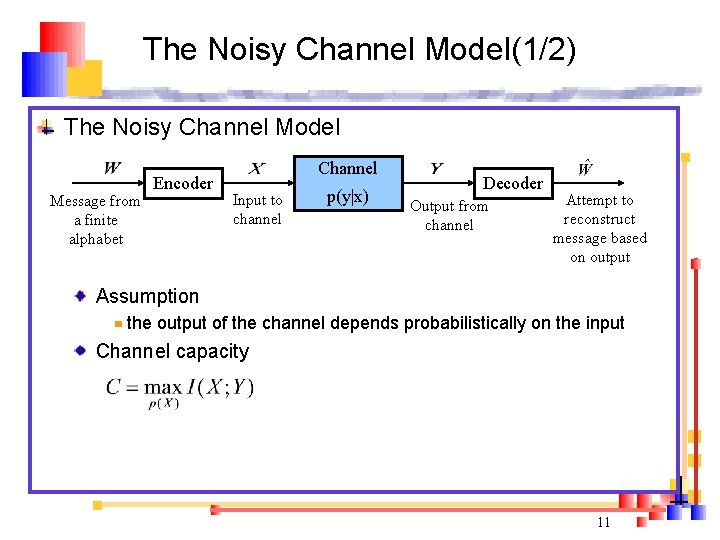The Noisy Channel Model(1/2) The Noisy Channel Model Message from a finite alphabet Encoder Channel Input to channel p(y|x) Decoder Output from channel Attempt to reconstruct message based on output Assumption the output of the channel depends probabilistically on the input Channel capacity 11The Noisy Channel Model(2/2) The Noisy Channel Model in Linguistics Noisy Channel Decoder decode the output to give the most likely input : language model, : channel probability applications MT, POS tagging, OCR, Speech recognition, . . . 12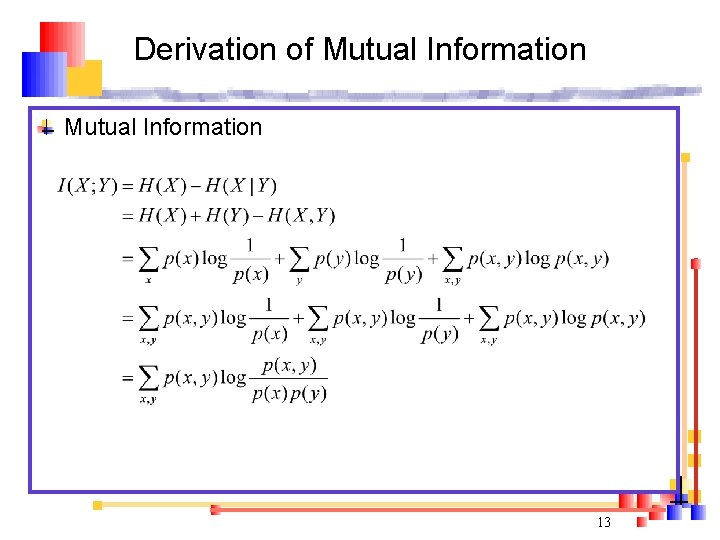Derivation of Mutual Information 13